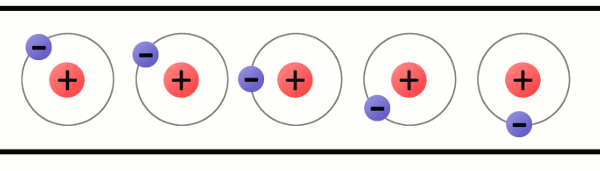Skip to main content

## Akoranga 11 Mahi Tuatahi

1. What are some common carriers of charge?

### Whakatika

• Electrons are the charge carriers in metals
• In solution, charged ions are the charge carriers
• Electrically charged gas in plasma

## Conductors

A conductor is a material through which charge can move freely.## Insulators

A material through which electrons cannot easily flow

• The charge carriers encounter a large friction force when trying to move
• This friction causes heat to build up
• The material can melt or catch fire if enough heat builds up!
• e.g. wood if current is applied

### Tūhura|Investigation

• Collect a powerpack + power cable
• 2x crocodile wires
• 1x resistor
• 1x pencil
1. Sharpen the pencil at both ends so the lead is exposed
2. Connect the two wires to the DC red/black plugs and then to either end of the resistor
3. Turn the voltage to 12V and switch on the power pack!
4. Turn off the power pack and then do the same thing with the pencil

## Current

• Current is the flow of charge (often electrons).
• It is the measure of the rate at which the charge flows (Amperes).
• Recall: $1C$ of charge is $6.25\times10^{18}$ electrons

\begin{aligned} & I = \frac{q}{t} \newline & \text{I = current measured in what?} \newline & \text{q = charge measure in what?} \newline & \text{t = time measured in what?} \end{aligned}

### Pātai

1. If $10A$ flows through a wire, how much charge passes a point in $5s$?
2. A total charge of $0.12C$ passes a point in $5s$. What is the current?
3. $0.02C$ of charge passed through a resistor in 1 minute. What was the current?
4. If the current is $0.3A$, how much charge will pass a point in 10 minutes?

### Conventional Current

• Back in the old days they thought current was the flow of positive charge, but these days we know it is the flow of negative charge
• This is because protons (positive) are fixed inside the nucleus of an atom and nuclei cannot move, whereas electrons (negative) can move freely in some materials

• Conventional current is the direction that positive charges move in a circuit. From the positive terminal to the negative terminal.
• However, electrons actually move from the negative terminal to the positive terminal, which is opposite to conventional current.